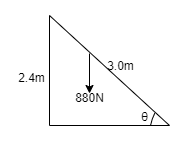# Problem: Tom is climbing a 3.0-m-long ladder that leans against a vertical wall, contacting the wall 2.4 m above the ground. His weight of 880 N is a vector pointing vertically downward. (Weight is measured in newtons, abbreviated N.)A. What is the magnitude of the component of Tom's weight parallel to the ladder?B. What is the magnitude of the component of Tom's weight perpendicular to the ladder?

###### FREE Expert Solution

We can visualize this problem in the diagram below:Sinθ = opposite/hypotenuse = 2.4/3.0

Cosθ = adjacent/hypotenuse = sqrt(3.02-2.42)/3.0

A.

From the figure, the magnitude of weight parallel to the ladder is:

95% (34 ratings)###### Problem Details

Tom is climbing a 3.0-m-long ladder that leans against a vertical wall, contacting the wall 2.4 m above the ground. His weight of 880 N is a vector pointing vertically downward. (Weight is measured in newtons, abbreviated N.)

A. What is the magnitude of the component of Tom's weight parallel to the ladder?

B. What is the magnitude of the component of Tom's weight perpendicular to the ladder?

Frequently Asked Questions

What scientific concept do you need to know in order to solve this problem?

Our tutors have indicated that to solve this problem you will need to apply the Trig Review concept. You can view video lessons to learn Trig Review. Or if you need more Trig Review practice, you can also practice Trig Review practice problems.

What professor is this problem relevant for?

Based on our data, we think this problem is relevant for Professor Anderson's class at SDSU.